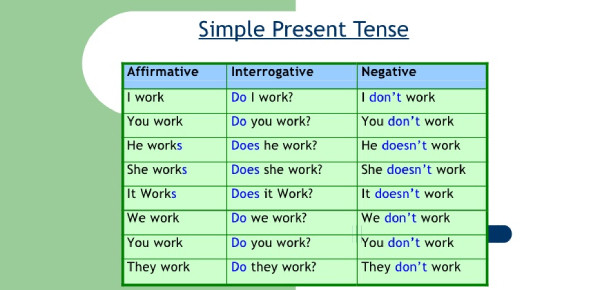# Simple Present Tense MCQs Quiz With Answers

Approved & Edited by ProProfs Editorial Team
At ProProfs Quizzes, our dedicated in-house team of experts takes pride in their work. With a sharp eye for detail, they meticulously review each quiz. This ensures that every quiz, taken by over 100 million users, meets our standards of accuracy, clarity, and engagement.
| Written by Ndutz
N
Ndutz
Community Contributor
Quizzes Created: 1 | Total Attempts: 17,317
Questions: 10 | Attempts: 17,390SettingsTake this simple present tense MCQs quiz with answers today if you need to hone your grammar skills in a quick and fun manner! This quiz contains questions in the form of fill-in-the-blanks on the simple past tense. Taking this quiz will not only help you in your practice, but also it might help you learn a thing or two about the usage of simple past tense in daily life and in tests too. Let's get down to it and dive right in!

• 1.

### I _____ (be) a student.

• A.

Are

• B.

Is

• C.

Am

C. Am
Explanation
The correct answer is "am" because the subject "I" is a singular pronoun, and the verb "be" should agree with the subject in number. In this case, "am" is the correct form of the verb "be" to use with the singular subject "I".

Rate this question:

• 2.

### My father __________ excuses when I feel like going to the cinema.

• A.

Make always

• B.

Always make

• C.

Always makes

B. Always make
Explanation
The correct answer is "always makes". In this sentence, the subject "my father" is singular, so the verb should also be singular. Therefore, "makes" is the correct form of the verb to match with "my father". The word "always" is an adverb that describes the frequency of the action, but it does not affect the verb form.

Rate this question:

• 3.

### She ________ (not, be) six years old.

• A.

Isn't

• B.

Are not

• C.

Not is

A. Isn't
Explanation
The correct answer is "isn't." This is the contraction of "is not," which is used to negate the statement. In this sentence, the subject "she" is being described as not being six years old.

Rate this question:

• 4.

### The flowers _______________ (be, normally) watered by Bob ....

• A.

Are normally

• B.

Normally are

• C.

Normally is

A. Are normally
Explanation
The correct answer is "are normally" because it follows the subject-verb agreement rule. In this sentence, "flowers" is the subject, which is plural, so the verb "are" should be used. Additionally, "normally" is an adverb that describes how the flowers are watered, so it should come after the verb "are".

Rate this question:

• 5.

### I ___________ (not, know) what you mean.

• A.

Doesn't know

• B.

Not know

• C.

Don't know

C. Don't know
Explanation
The correct answer is "don't know". In this sentence, the subject "I" is followed by the verb "don't know" which is the correct form of the verb "know" in the negative form. The sentence is expressing the speaker's lack of knowledge or understanding about something.

Rate this question:

• 6.

### They study math two times a weeks.

• A.

True

• B.

False

A. True
Explanation
The given statement is "They study math two times a week." The correct answer is True because it indicates that "they" study math twice a week.

Rate this question:

• 7.

### My mother are dentist

• A.

True

• B.

False

B. False
Explanation
The given sentence "My mother are dentist" is grammatically incorrect. The verb "are" is used with plural subjects, while the subject "my mother" is singular. The correct form should be "My mother is a dentist." Therefore, the correct answer is False.

Rate this question:

• 8.

### He don't know  what I mean

• A.

True

• B.

False

B. False
Explanation
The given statement is grammatically incorrect. The correct form should be "He doesn't know what I mean." Therefore, the correct answer is False.

Rate this question:

• 9.

### I was a teacher now.

• A.

True

• B.

False

B. False
Explanation
The statement "I was a teacher now" is grammatically incorrect. The word "now" suggests that the person is currently a teacher, but the use of the past tense "was" contradicts that. Therefore, the correct answer is False.

Rate this question:

• 10.

### She always eats vegetables every day

• A.

True

• B.

FalseBack to top